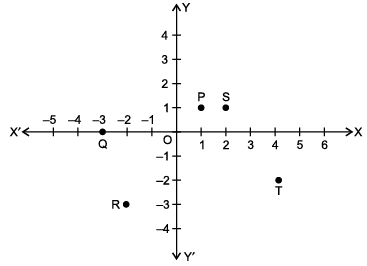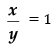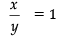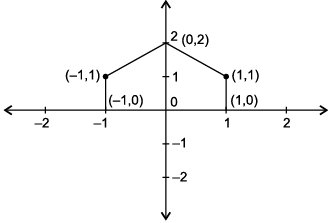Test: Coordinate Geometry- Case Based Type Questions

# Test: Coordinate Geometry- Case Based Type Questions - Class 9

Test Description

## 10 Questions MCQ Test Mathematics (Maths) Class 9 - Test: Coordinate Geometry- Case Based Type Questions

Test: Coordinate Geometry- Case Based Type Questions for Class 9 2023 is part of Mathematics (Maths) Class 9 preparation. The Test: Coordinate Geometry- Case Based Type Questions questions and answers have been prepared according to the Class 9 exam syllabus.The Test: Coordinate Geometry- Case Based Type Questions MCQs are made for Class 9 2023 Exam. Find important definitions, questions, notes, meanings, examples, exercises, MCQs and online tests for Test: Coordinate Geometry- Case Based Type Questions below.
Solutions of Test: Coordinate Geometry- Case Based Type Questions questions in English are available as part of our Mathematics (Maths) Class 9 for Class 9 & Test: Coordinate Geometry- Case Based Type Questions solutions in Hindi for Mathematics (Maths) Class 9 course. Download more important topics, notes, lectures and mock test series for Class 9 Exam by signing up for free. Attempt Test: Coordinate Geometry- Case Based Type Questions | 10 questions in 20 minutes | Mock test for Class 9 preparation | Free important questions MCQ to study Mathematics (Maths) Class 9 for Class 9 Exam | Download free PDF with solutions
 1 Crore+ students have signed up on EduRev. Have you?
Test: Coordinate Geometry- Case Based Type Questions - Question 1

### Five friends playing a game in which they are standing at different positions, P, S, T, R andRohan is watching them playing. Few questions came to Rohan's mind while watching the game. Give answer to his questions by looking at the figure.Q. Name the point whose y-co-ordinate is zero :

Detailed Solution for Test: Coordinate Geometry- Case Based Type Questions - Question 1
As point Q lies on x-axis. Therefore, its y-coordinate is zero.
Test: Coordinate Geometry- Case Based Type Questions - Question 2

### Five friends playing a game in which they are standing at different positions, P, S, T, R andRohan is watching them playing. Few questions came to Rohan's mind while watching the game. Give answer to his questions by looking at the figure.Q. Name the point lying in the third quadrant.

Detailed Solution for Test: Coordinate Geometry- Case Based Type Questions - Question 2
In third quadrant, both x-co-ordinate and y-coordinate are negative.
Test: Coordinate Geometry- Case Based Type Questions - Question 3

### Five friends playing a game in which they are standing at different positions, P, S, T, R andRohan is watching them playing. Few questions came to Rohan's mind while watching the game. Give answer to his questions by looking at the figure.Q. What are the coordinates of P?

Detailed Solution for Test: Coordinate Geometry- Case Based Type Questions - Question 3
The Coordinate of P is (1, 1)
Test: Coordinate Geometry- Case Based Type Questions - Question 4

Five friends playing a game in which they are standing at different positions, P, S, T, R andRohan is watching them playing. Few questions came to Rohan's mind while watching the game. Give answer to his questions by looking at the figure.

Q. Name the polygon formed on joining all these five points in an order.

Detailed Solution for Test: Coordinate Geometry- Case Based Type Questions - Question 4
Pentagon (5 sided polygon).
Test: Coordinate Geometry- Case Based Type Questions - Question 5

Five friends playing a game in which they are standing at different positions, P, S, T, R andRohan is watching them playing. Few questions came to Rohan's mind while watching the game. Give answer to his questions by looking at the figure.

Q. (x, y) = (y, x), if :

Detailed Solution for Test: Coordinate Geometry- Case Based Type Questions - Question 5Since,⇒ x = y

Also, (x, y) = (y, x), if x = y

Test: Coordinate Geometry- Case Based Type Questions - Question 6

Sohan draws a gate of a temple on the graph paper. He has following points :

(– 1, 0), (1, 0), (1, 1), (– 1, 1) and (0, 2)Q. In which quadrant (– 1, 1) lies ?

Detailed Solution for Test: Coordinate Geometry- Case Based Type Questions - Question 6

In 2nd quadrant, x-co-ordinate is negative and y-coordinate is positive.

Test: Coordinate Geometry- Case Based Type Questions - Question 7

Sohan draws a gate of a temple on the graph paper. He has following points :

(– 1, 0), (1, 0), (1, 1), (– 1, 1) and (0, 2)Q. Write the abscissa of the point (0, 2).

Detailed Solution for Test: Coordinate Geometry- Case Based Type Questions - Question 7
x-co-ordinate of a point also called abscissa.
Test: Coordinate Geometry- Case Based Type Questions - Question 8

Sohan draws a gate of a temple on the graph paper. He has following points :

(– 1, 0), (1, 0), (1, 1), (– 1, 1) and (0, 2)Q. Name the closed figure obtained.

Detailed Solution for Test: Coordinate Geometry- Case Based Type Questions - Question 8
A pentagon has five sides.
Test: Coordinate Geometry- Case Based Type Questions - Question 9

Sohan draws a gate of a temple on the graph paper. He has following points :

(– 1, 0), (1, 0), (1, 1), (– 1, 1) and (0, 2)Q. Write the ordinate of the point (1, 0).

Detailed Solution for Test: Coordinate Geometry- Case Based Type Questions - Question 9
y-co-ordinate of a point also called ordinate.
Test: Coordinate Geometry- Case Based Type Questions - Question 10

Sohan draws a gate of a temple on the graph paper. He has following points :

(– 1, 0), (1, 0), (1, 1), (– 1, 1) and (0, 2)Q. Which point from the following lies on Y-axis ?

Detailed Solution for Test: Coordinate Geometry- Case Based Type Questions - Question 10
On Y-axis, x-co-ordinate is zero.

## Mathematics (Maths) Class 9

42 videos|378 docs|65 tests
Information about Test: Coordinate Geometry- Case Based Type Questions Page
In this test you can find the Exam questions for Test: Coordinate Geometry- Case Based Type Questions solved & explained in the simplest way possible. Besides giving Questions and answers for Test: Coordinate Geometry- Case Based Type Questions, EduRev gives you an ample number of Online tests for practice

## Mathematics (Maths) Class 9

42 videos|378 docs|65 tests